# Samacheer Kalvi 8th Maths Book Solutions Term 1 Chapter 4 | Geometry Answers For Tamil Nadu Board

Samacheer Kalvi 8th Maths Book Solutions Term 1 Chapter 4 – Geometry is available here. The Samacheer Kalvi 8th Maths book answers of Term 1 Chapter 4, available at BYJU’S, contain step by step explanations designed by our Mathematics experts. All these important questions are based on the new pattern prescribed by the Tamil Nadu board. Students can also get the solutions of other chapters on Samacheer Kalvi 8th Maths solutions. Here, in this article below please find the Samacheer Kalvi Class 8 Maths Book Term 1 Chapter 4 Questions with Solutions that we have compiled for the students to solve and practice.

## Samacheer Kalvi 8th Class Maths Textbook Term 1 Chapter 4 Questions and Solutions

Term 1 Chapter 4 of the Samacheer Kalvi 8th Maths guide will help the students to solve problems related to similar triangles, congruent triangles, construction of quadrilaterals.

### Samacheer Kalvi 8th Maths Term 1 Chapter 4: Geometry Book Exercise 4.1 Questions and Solutions

Question 1: From the given figure, prove that △ABC ~ △DEF.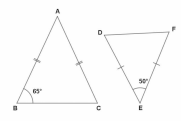Solution:

From the △ABC,

AB = AC

It is an isosceles triangle.

Angles opposite to equal sides are equal.

∠B = ∠C = 65o

∠B + ∠C = 65o + 65o = 130o

Sum of 3 angles of a triangle = 180o

∠A + ∠B + ∠C = 180o

∠A + 130o = 180o

∠A = 180o – 130o

∠A = 50o

From the △DEF, ∠D = 50o

Sum of the remaining angles = 180o – 50o = 130o

DE = FD

∠D = ∠F

From △ABC and △DEF,

∠E = 130o / 2 = 65o

∠A = ∠D = 50o

∠B = ∠E = 65o

∠C = ∠F = 65o

By AAA criterion, △ABC ~ △DEF.

Question 2: In the given figure, if △EAT ~ △BUN, find the measure of all angles.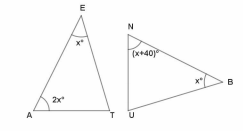Solution:

Given that △EAT ~ △BUN,

Corresponding angles are equal,

∠E = ∠B — (1)

∠A = ∠U — (2)

∠T = ∠N — (3)

∠E = xo

∠A = 2xo

Sum of three angles of a triangle = 180o

In △EAT,

x + 2x + ∠T = 180o

∠T = 180o – 3xo — (4)

Also in △BUN,

x + 40o + x + ∠U = 180o

2x + 40o + ∠U = 180o

∠U = 140o – 2xo

Now by (2)

∠A = ∠U

2x = 140 – 2x

4x = 140

x = 35o

∠A = 2x = 2 * 35 = 70o = ∠U

∠N = x + 40o = 35 + 40o = 75o

∠E = 35o = ∠U

Question 3: In the given figure, D is the midpoint of OE and ∠CDE = 90°. Prove that △ODC ≡ △EDC.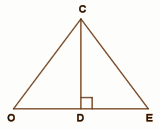Solution:

 Serial number Statement Reason 1 OD = ED D is the midpoint of OE 2 DC = DC Common side 3 ∠CDE = ∠CDO Linear pair and given ∠CDE = 90° 4 △ODC ≡ △EDC By RHS criteria

### Samacheer Kalvi 8th Maths Term 1 Chapter 4: Geometry Book Exercise 4.2 Questions and Solutions

Question 1: The height of a tower is measured by a mirror on the ground at R by which the top of the tower’s reflection is seen. Find the height of the tower.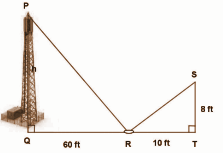Solution:

The image and its reflection make similar shapes.

△PQR ~ △STR,

PQ / ST = QR / TR = PR / SR

PQ / ST = QR / TR

h / 8 = 60 / 10

h = [60 / 10] * 8

= 48 feet

Height of the tower = 48 feet

Question 2: In the figure, given that ∠1 = ∠2 and ∠3 = ∠4. Prove that △MUG ≡ △TUB.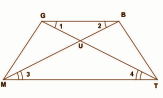Solution:

 Serial number Statement Reason 1 In △MUG and △TUB, MU = TU ∠3 = ∠4, opposite sides of equal angles 2 UG = UB ∠1 = ∠2, the side opposite to equal angles are equal 3 ∠GUM = ∠BUT Vertically opposite angles 4 △MUG ≡ △TUB By SAS criteria

Question 3: If △WAR ≡ △MOB, name the additional pair of corresponding parts. Name the criterion used by you.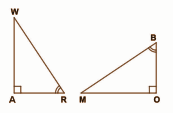Solution:

Given: △WAR ≡ △MOB,

∠RWA ≡ ∠BMO [Sum of three angles of a triangle is 180o]

The criteria used here is the angle sum property of triangles.

### Samacheer Kalvi 8th Maths Term 1 Chapter 4: Geometry Book Exercise 4.3 Questions and Solutions

Question 1: PLAY, PL= 7 cm, LA = 6 cm, AY= 6 cm, PA = 8 cm and LY = 7 cm.

Solution: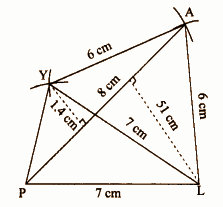Steps of construction:

1] A line segment PL = 7cm is drawn.

2] Two arcs of radii 8cm and 6cm are drawn by taking P and L as centres. Both the arcs cut at A.

3] Join PA and LA.

4] Two arcs of radii 7cm and 6cm are drawn by taking A and L as centres. Both the arcs cut at Y.

5] Join LY, PY and AY.

6] The required quadrilateral is PLAY.

Question 2: LIKE, LI = 4.2 cm, IK = 7 cm, KE = 5 cm, LK = 6 cm and IE = 8 cm.

Solution: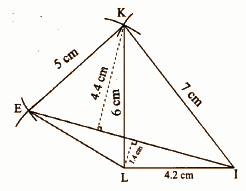Steps of construction:

1] A line segment LI = 4.2 cm is drawn.

2] Two arcs of radii 7cm and 6cm are drawn by taking I and L as centres. Both the arcs cut at K.

3] Join LK and IK.

4] Two arcs of radii 8cm and 5cm are drawn by taking I and K as centres. Both the arcs cut at E.

5] Join LE, IE and KE.

6] The required quadrilateral is LIKE.

Question 3: PQRS, PQ = QR = 3.5 cm, RS = 5.2 cm, SP = 5.3 cm and ∠Q = 120o.

Solution: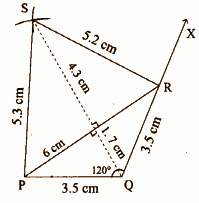Steps of construction:

1] A line segment PQ = 3.5 cm is drawn.

2] ∠Q = 120o is made. Ray QX is drawn.

3] An arc of radius 3.5cm is drawn with Q as the centre and it cuts the ray QX at R.

4] Two arcs of radii 5.2cm and 5.5cm are drawn with R and P as centres which cuts at S.

5] Join PS and RS.

6] The required quadrilateral is PQRS.